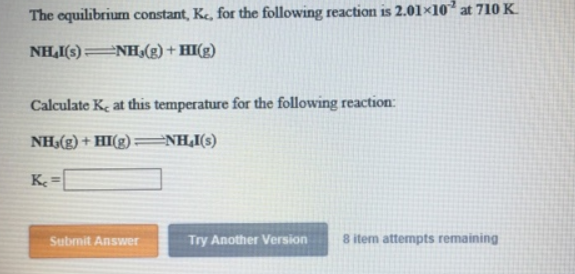# Problem: The equilibrium constant, Kc, for the following reaction is 2.01 x 10-2 at 710 K. NH4I (s) ⇌ NH3 (g) + HI (g) Calculate Kc at this temperature for the following reaction: NH3 (g) + HI (g) ⇌ NH4I (s)

###### FREE Expert Solution
97% (265 ratings)###### Problem Details

The equilibrium constant, Kc, for the following reaction is 2.01 x 10-2 at 710 K.

NH4I (s) ⇌ NH3 (g) + HI (g)

Calculate Kat this temperature for the following reaction:

NH3 (g) + HI (g) ⇌ NH4I (s)